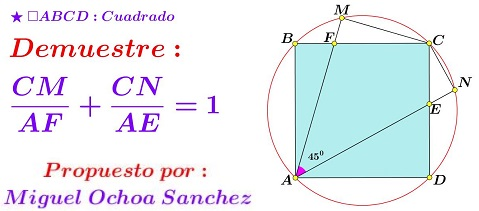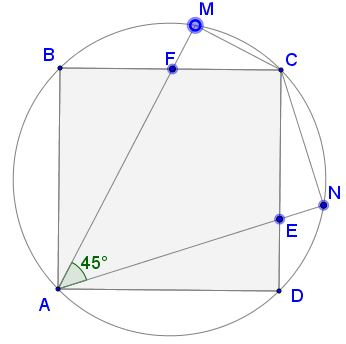# Geometric/Trigonometric Observation/Exercise

### Source### Problem

Let $M\,$ be a point on the minor arc $\overset{\frown}{BC},\,$ of the circumcircle $(ABCD)\,$ of the square $ABCD;\,$ let $N\,$ lie on the minor arc $\overset{\frown}{CD}\,$ such that $\angle MAN=45^{\circ}.\,$Define $E\,$ and $F\,$ as the intersections of $AN\,$ with $CD\,$ and $AM\,$ with $BC,\,$ respectively. Prove that

$\displaystyle \frac{CM}{AF}+\frac{CN}{AE}=1.$

### Solution 1

Simple angle chasing shows that $\angle BAF=\angle FCM\,(=\alpha)\,$ and $\angle DAE=\angle ECN\,(=\beta)\,$ and, in addition, $\alpha+\beta=45^{\circ}.$With $AC\,$ being a diameter of $(ABCD),\,$ triangles $ACM\,$ and $ACN\,$ are right. We have

From $\Delta ABF,\,$ $AF=\displaystyle \frac{AB}{\cos\alpha},$
from $\Delta ACM,\,$ $CM=AC\cdot\sin\beta,$
from $\Delta ADE,\,$ $AE=\displaystyle \frac{AD}{\cos\beta},$ and
from $\Delta ACN,\,$ $CN=AC\cdot\sin\alpha.$

Also, $AB=AD\,$ and $AC=\sqrt{2}\cdot AB.\,$ Assume $AB=1.\,$ It follows that

\displaystyle\begin{align}\frac{CM}{AF}+\frac{CN}{AE}&=\sqrt{2}\cdot\sin\beta\cos\alpha+\sqrt{2}\cdot\sin\alpha\cos\beta\\ &=\sqrt{2}\sin(\alpha+\beta)=\sqrt{2}\sin 45^{\circ}=1. \end{align}

### Solution 2

Let the side of the square be $s$, $\angle CAM=\alpha$ and $\angle CAN=\beta$. Thus, $\beta=45^o-\alpha$, $\angle BAF=45^o-\alpha$ and $\angle AFB=45^o+\alpha.\,$ Applying sine rule to triangles $ACM$ and $AFB$,

$\displaystyle \frac{1}{s\sqrt{2}}=\frac{\sin\alpha}{CM},~\frac{\sin(45^o+\alpha)}{s}=\frac{1}{AF}.$

Dividing one of the the equations by the other and rearranging,

\displaystyle\begin{align} \frac{CM}{AF}&=\sqrt{2}\sin\alpha\sin(45^o+\alpha)=\sqrt{2}\sin\alpha\cos(45^o-\alpha), \\ \frac{CN}{AE}&=\sqrt{2}\sin\beta\cos(45^o-\beta)=\sqrt{2}\sin(45^o-\alpha)\cos\alpha.~\text{(Analogy)} \end{align}

Thus,

$\displaystyle \frac{CM}{AF}+\frac{CN}{AE}=\sqrt{2}\left[\sin\alpha\cos(45^o-\alpha)+\sin(45^o-\alpha)\cos\alpha\right]=\sqrt{2}\sin 45^o = 1.$

### Solution 3

We shall refer to the following configuration:$\Delta ASC\sim\Delta ACT,\,$ hence,

(1)

$\displaystyle \frac{AC}{AT}=\frac{AS}{AC}.$

Further, $\angle SAC=45^{\circ},\,$ $F\,$ is the orthocenter of $\Delta ASC\,$ such that $AF=CS.\,$ Similarly, in $\Delta ACT,\,$ $AE=CT.$

(2)

$\displaystyle\Delta AMC\sim\Delta ANT\;\Rightarrow\;\frac{CM}{AC}=\frac{AE-CN}{AT}$

(3)

$\displaystyle\Delta ANC\sim\Delta AMS\;\Rightarrow\;\frac{CN}{AC}=\frac{AF-CM}{AS}$

(1)-(3) yield

(4)

$\displaystyle\frac{CM}{AE-CN}\frac{AF-CM}{CN}.$

And, after a sequence of calculations,

$AE\cdot CM+AF\cdot CN=AE\cdot AF,$

so that $\displaystyle \frac{CM}{AF}+\frac{CN}{AE}=1.$

### Acknowledgment

The above problem has been posted by Miguel Ochoa Sanchez was originally posted at the Peru Geometrico facebook group and appeared on my timeline. Solution 2 is by Amit Itagi; Solution 3 is by Thanos Kalogerakis.

• Angle Trisectors on Circumcircle
• Equilateral Triangles On Sides of a Parallelogram
• Pompeiu's Theorem
• Pairs of Areas in Equilateral Triangle
• The Eutrigon Theorem
• Equilateral Triangle in Equilateral Triangle
• Seven Problems in Equilateral Triangle
• Spiral Similarity Leads to Equilateral Triangle
• Parallelogram and Four Equilateral Triangles
• A Pedal Property in Equilateral Triangle
• Miguel Ochoa's van Schooten Like Theorem
• Two Conditions for a Triangle to Be Equilateral
• Incircle in Equilateral Triangle
• When Is Triangle Equilateral: Marian Dinca's Criterion
• Barycenter of Cevian Triangle
• Excircle in Equilateral Triangle
• Converse Construction in Pompeiu's Theorem
• Wonderful Trigonometry In Equilateral Triangle
• 60o Angle And Importance of Being The Other End of a Diameter
• One More Property of Equilateral Triangles
• Van Khea's Quickie
• Equilateral Triangle from Three Centroids
•